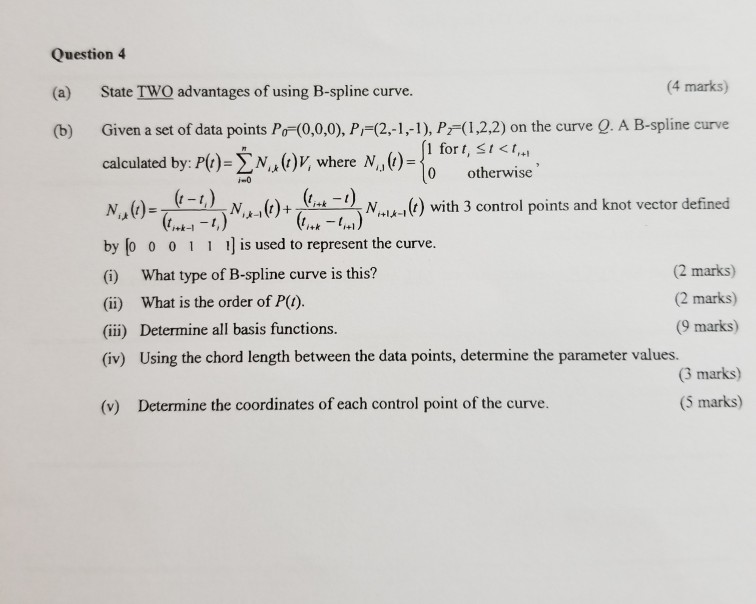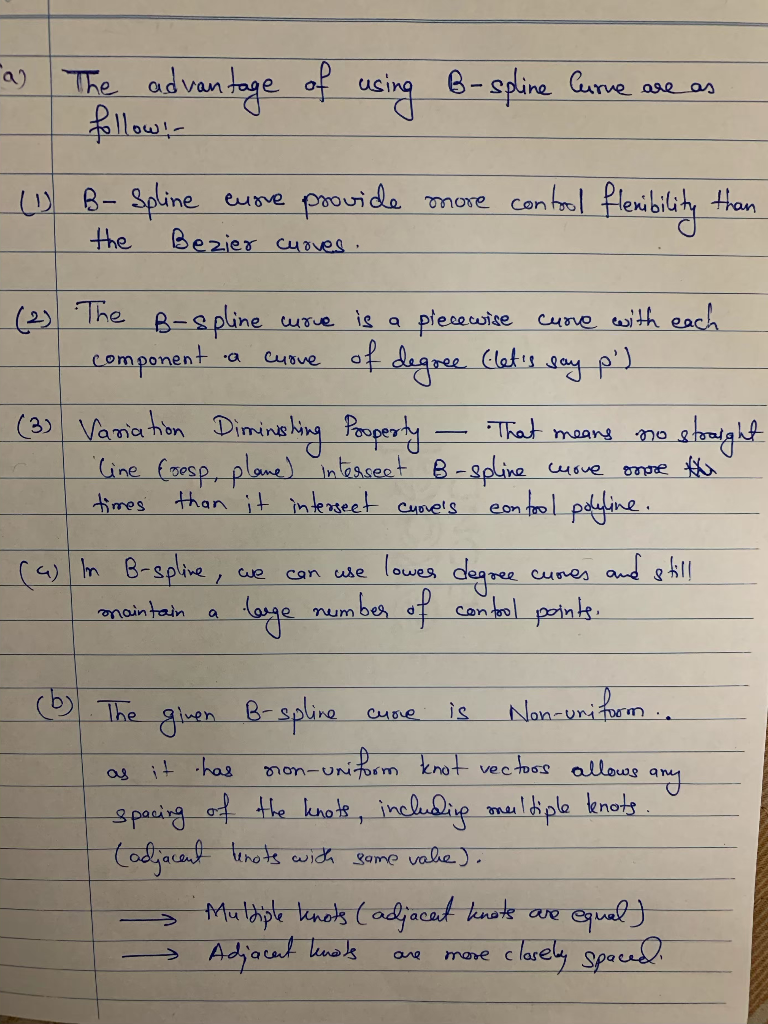# Question 4 (4 marks) (a) State TWO advantages of using B-spline curve (b) Given a set of data points Po- (0,0,0), P(...Question 4 (4 marks) (a) State TWO advantages of using B-spline curve (b) Given a set of data points Po- (0,0,0), P(2,-1,-1), P-(1,2.2) on the curve Q. A B-spline curve calculated by: P(,)-Σ N,,0)I, where N,,0-11 fort, sl

.(c) The order of P(t) is 2

##### Add Answer of: Question 4 (4 marks) (a) State TWO advantages of using B-spline curve (b) Given a set of data points Po- (0,0,0), P(...
More Homework Help Questions Additional questions in this topic.